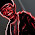## Friday, 24 August 2012

### CBSE Class 10 - Maths - CH8 - Introduction to Trigonometry

Introduction to TrigonometryHipparchus: Father of Trignometry Image credits:wikipedia

Q1: If cot A=12/5, then the value of  (sin A + cos A) × cosec A is :
(a) 13/5
(b) 17/5
(c) 14/5
(d) 1

Q2: cos 1° × cos 2° × cos 3° × ... × cos 180° is equal to :
(a)1
(b) 0
(c) 1/2
(d) –1

Q3: If sin θ = cos θ, then value of θ is
(a) 0°
(b) 45°
(c) 30°
(d) 90°

Q4: Which of the following is correct some θ such that 0° ≤ θ < 90°
(a) 1/secθ > 1
(b) 1/secθ < 1
(c)  secθ = 0
(d) 1/cosθ < 1

Q5: sin θ = 4/3 for some angle θ, is :(a) true
(b) false
(c) may be true for certain cases
(d) none of these

Q6: If sin θ = cos θ, then the value of cosec θ is :
(a) 2
(b) 1
(c) 2/√3
(d) √2

Q7: The value of sin18°/cos72° is :(a) 1
(b) 0
(c) –1
(d) 1/2

Q8: Given that sin A=1/2 and cos B=1/√2 then the value of (A + B) is:
(a) 30°
(b) 45°
(c) 75°
(d) 15°

Q9: If sec θ + tan θ = x, then tan θ is:
(a) (x2 + 1)/x
(b) (x2 - 1)/x
(c) (x2 + 1)/2x
(d) (x2 - 1)/2x

Q10: If sec θ – tan θ = 1/3, the value of (sec θ + tan θ)
(a) 1
(b) 2
(c) 3
(d) 4

Q11: 9sec2 θ - 9tan2 θ  is equal to :
(a) 1
(b) –1
(c) 9
(d) –9

Q12: The value of tan 1°.tan 2°.tan 3° ... tan 89° is :
(a) 0
(b) 1
(c) 2
(d) ½

Q13: The value of [(cos A/cot A)  + sin A] is:
(a) cot A
(b) 2 sin A
(c) 2 cos A
(d) sec A

Q14: If tan 2A = cot (A – 18°), then the value of A is:
(a) 18°
(b) 36°
(c) 24°
(d) 27°

Q15: if 5 tan θ = 4, then value of (5 sin θ - 4 cos θ)/(5 sin θ + 4 cos θ) is:
(a) 5/3
(b) 0
(c) 5/6
(d) 1/6

Q16: If cos 3θ = √3/2; 0 < θ < 20°, then the value of  θ is :
(a) 15°
(b) 10°
(c) 0°
(d) 12°

Q17: In sin 3θ = cos (θ – 26°), where 3θ and (θ – 26°) are acute angles, then value of θ is :
(a) 30°
(b) 29°
(c) 27°
(d) 26°

Q18: sin (60° + θ) – cos (30° – θ) is equal to :
(a) 2 cos θ
(b) 2 sin θ
(c) 0
(d) 1

1:(b) 17/5
[Hint: (sin A + cos A) × cosec A = 1 + cot A = 1 + 12/5 = 17/5]

2. (b) 0   (cos 90° = 0)

3. (b) 45°

4. (b) 1/secθ < 1    [Hint: 1/secθ = cosθ. And value of cosθ ranges from 0 to 1)]

5. (b) false [Hint: sin θ ranges from 0 to 1. It can't be greater than 1]

6. (d) √2  [Hint: cosec 45° = √2]

7.  (a) [Hint: sin18°/cos72° = sin(90°-72°)/cos72°=cos72°/cos72° = 1]

8. (c) 75° [Hint:sin A = sin 30 = 1/2 ⇒ A = 30° and cos B = cos 45° = 1/√2 ⇒ B = 45°]

9. (d) (x2 - 1)/2x
[Hint: Given sec θ + tan θ = x.               ...(I)
Also sec2 θ - tan2 θ = 1
⇒ (sec θ + tan θ)(sec θ - tan θ) = 1
⇒ sec θ - tan θ = 1/x                 ...(II)
Divide II from I,
sec θ + tan θ - (sec θ - tan θ) = x -1/x
⇒ 2tan θ = (x2 - 1)/x
⇒ tan θ = (x2 - 1)/2x]

10. (c) 3   [Hint: Use sec2 θ - tan2 θ = 1]

11: (c) 9  [Hint: Use sec2 θ - tan2 θ = 1]

12: (b) 1
[Hint: tan θ. cot θ = 1, tan(90 - θ) = cot θ  and tan45° = 1
Given  tan 1°.tan 2°.tan 3° ... tan 88°. tan 89°
= (tan 1°. tan 89°).(tan 2°.tan 88°) ... (tan44°.tan46°)(tan45°)
=  [tan 1°. tan(90°-1°)].[tan2°.tan(90°-2)] ... [(tan44°.tan(90-44)].1
=  (tan 1°. cot 1°).(tan 2°.cot2°) ... (tan44°.cot44°)
= 1.1 ... 1 = 1]

13: (b) 2 sin A [Hint: Use cot A = cos A/sin A]

14: (b) 36°
[Hint: Given, tan 2A = cot (A – 18°)
⇒ tan 2A = tan(90 - (A – 18°))
⇒ tan 2A =  tan (108° – A)
⇒ 2A = 108° – A
⇒ 3A = 108°
⇒ A = 36°]

15:  (b) 0
[Hint:  Divide both numerator and denominator by cos θ and solve]

16: (b) 10° [Hint: cos 30° = √3/2]

17: (b) 29°
[Hint: sin 3θ = cos (θ – 26°)
⇒ sin 3θ = sin {90°– (θ – 26°)}
⇒ 3θ= 90° – θ+ 26° ⇒ θ= 116°/4
⇒ θ = 29°]

18: (c) 0
[Hint: sin (90° – α) = cos α
sin(60° + θ) – cos (30° – θ) = cos (90 -(60° + θ)) - cos (30° – θ) = cos (30° – θ) - cos (30° – θ) = 0]1.not very difficult questions

2.This comment has been removed by the author.

1.3.ya,i need some prove Qs
thanks

4.there should be some solved theorems

5.more questions should be there.....

6.thanx for these question

7.nice questions....but more questions should be there..

8.More questions needs to be there....

9.More questions needs to be there....

10.This comment has been removed by the author.

11.Thank you !
Can you provide more mcq on trigonometry.

12.yes this is a not diffcult queston

13.